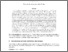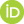# Semi-formally stated open problems on computable sets X={n \in N: \phi(n)}, where \phi(n) has the same intuitive meaning for every n \in N and the finiteness (infiniteness) of X remains conjectured

Kurpaska, Sławomir and Tyszka, Apoloniusz (2019) Semi-formally stated open problems on computable sets X={n \in N: \phi(n)}, where \phi(n) has the same intuitive meaning for every n \in N and the finiteness (infiniteness) of X remains conjectured. [Preprint]Preview
Text
semi_formally.pdf

## Abstract

Let b=((24!)!)!, and let P_{n^2+1} denote the set of all primes of the form n^2+1. Let M denote the set of all positive multiples of elements of the set P_{n^2+1} \cap (b,\infty). The set X={0,...,b} \cup M satisfies the following conditions: (1) card(X) is greater than a huge positive integer and it is conjectured that X is infinite, (2) we do not know any algorithm deciding the finiteness of X, (3) a known and short algorithm for every n \in N decides whether or not n \in X, (4) a known and short algorithm returns an integer n such that X is infinite if and only if X contains an element greater than n. The following problem is open: define a set X \subseteq N such that X satisfies conditions (1)-(4) and a known and simple formula \phi(x) satisfies X={n \in N: \phi(n)}, where \phi(n) has the same intuitive meaning for every n \in N (5). The statements \phi(n) in condition (5) have always the same intuitive meaning, if the predicate \phi(x) expresses a natural property, the term propounded by the philosopher David Lewis (1941-2001). Let f(3)=4, and let f(n+1)=f(n)! for every integer n \geq 3. For an integer n \geq 3, let \Psi_n denote the following statement: if a system of equations S \subseteq {x_i!=x_{i+1}: 1 \leq i \leq n-1} \cup {x_i \cdot x_j=x_{j+1}: 1 \leq i \leq j \leq n-1} has only finitely many solutions in positive integers x_1,...,x_n, then each such solution (x_1,...,x_n) satisfies x_1,...,x_n \leq f(n). We prove that for every statement \Psi_n the bound f(n) cannot be decreased. The author's guess is that the statements \Psi_3,...,\Psi_9 are true. We prove that the statement \Psi_9 implies that the set X of all non-negative integers n whose number of digits belongs to P_{n^2+1} satisfies conditions (1)-(5).

 Export/Citation: EndNote | BibTeX | Dublin Core | ASCII/Text Citation (Chicago) | HTML Citation | OpenURL
 Social Networking:

Item Type: Preprint
Creators:
CreatorsEmailORCIDTyszka, Apoloniuszrttyszka@cyf-kr.edu.pl0000-0002-2770-5495
Additional Information: a shorter version was submitted to Logic and Logical Philosophy
Keywords: computable set X \subseteq N whose finiteness remains conjectured; computable set X \subseteq N whose infiniteness remains conjectured; David Lewis's notion of a natural property; intuitive meaning of a mathematical formula; Zenkin's super-induction
Subjects: Specific Sciences > Mathematics > Foundations
Specific Sciences > Mathematics > Logic
Depositing User: Apoloniusz Tyszka
Date Deposited: 02 Jan 2020 23:45
Item ID: 16775
Subjects: Specific Sciences > Mathematics > Foundations
Specific Sciences > Mathematics > Logic
Date: 31 December 2019
URI: http://philsci-archive.pitt.edu/id/eprint/16775

### Monthly Views for the past 3 yearsView Item